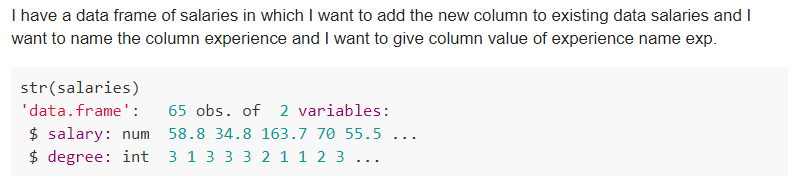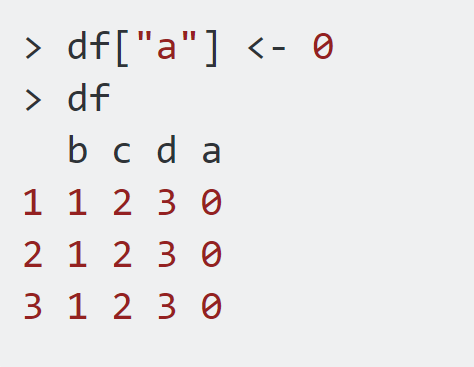# How To Add Column To DataFrame In R [Examples Included]

Here at HdfsTutorial, we keep on adding the subjective topics which can make your basic right. Earlier, we have talked about dataframe in R but many questions came for a dedicated topic to discuss about how to add column to dataframe R. And so, here is a detailed guide on how to add a new column to dataframe R.

##What is a dataframe?

As we know dataframe is a way to store the data in R. You can load a file to R and their data will be stored in the form of dataframe. We majorly load the data in the form of a dataframe so that the operation will be easier. There are a lot of inbuilt functions and operations which can be done directly on the dataframe. And this is one of the reasons why dataframe is so popular.

If you want to learn R, you can check our YouTube channel. You will get a free 8 hours of course on R.

As we all know, dataframe in R can be created using the function data.frame()

For example, if I have to create a dataframe with three vectors, I can create like below-

```n=c(2,3,5)
s=c(“aa”,”bb”,”cc”)
b=c(TRUE,FALSE,TRUE)
#create a dataframe using vectors
df=data.frame(n,s,b)
```

And this will give me an output like below-As you can see above, all the three vectors have been combined to create a dataframe which has 3 columns and 3 rows. Now there may be a requirement to add column to dataframe in R. This can be a business requirement to add a new column to dataframe R or maybe for your calculation.

## Why need to add new column to dataframe in R?

There can be various reason why you need to add a new column to dataframe R. Some of those can be like below-

• You got a data first and you stored in a dataframe and later due to some change in requirement, you have to add a new column. You can accomplish this with the help of r add column to dataframe.
• There might be some requirements where you need to add a custom column something like interest rate or total amount. In this case, also, you can add column to dataframe in R.

Similar to this, there can be various such requirements to add a new column to dataframe r. Now let’s see how to add a column to a dataframe in r.

## How to add a column to a dataframe R?

We can add a add column to dataframe. The condition is length should be the same.

That means, if you have a dataframe with 3 rows and 3 columns and you want to add a 4th column then the 4th column must have 3 records in it. Else you will be getting error.

Example:

Let’s say you want to add a new column to the above dataframe df with the name “new_col”. As we have 3 rows and so we need to have 3 records in the new column in this new_col column as well. So, we can define it like-

```df\$new_col=c(10,11,12)
print(df)```

As you can see I have added only 3 elements to this column.

Now in this dataframe, we have 4 columns and 3 rows.

Let’s try adding a new column to dataframe and take 4 elements-So, you can see I tried adding a new column with the name “new_col_2” to this existing dataframe with 4 elements and it has started showing error.

## How to add a new column to a dataframe in r Example

I found various questions on r add column to dataframe in a different forum and I am answering all those here.

### Question-1: Add new column to existing dataframe in RThe solution is fairly simple. Either we can use the above technique and write the code like-

```salaries\$experience=exp
```

Or we can also take the help of function cbind() to add a new column to dataframe in R.

```experience=exp
cbind(salaries, experience)
```

Both works for a similar purpose.

### Question-2: How to add new column to the dataframe in R at the start?As you might have experienced when we add new column to the dataframe r, it gets added at the end of the dataframe. But what if we want to add a new column to dataframe but at the start of the dataframe. This means we want the new column to be the 1st column.

Yes, we can do that. Let’s see how we can do that-

Let’s add a new column “a” to this dataframe-Now to take the column “a” at the 1st place, we have two options-

1. Sort the column by using the column name. As we have column names like a,b,c, and d and so if we will sort by column name then-new column “a” will come at first.But this technique might not work if your column names are not in such order. For example, if the column names are – a,b,z,x,f, and the newly added column is “c” then sorting by column name may not work. And in such cases, we can use the 2nd technique.
2. Here we can sort column by index like below-This way you can add a new column to the existing dataframe and also keep a new column at the start.

#### Conclusion

Using the above steps, you can add a new column to an existing dataframe in R and can also take it to the first place.

Do try and comment below if you find any difficulty.